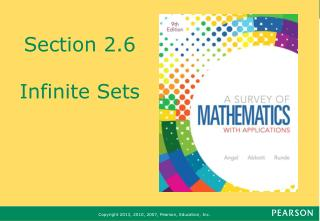DownloadDownload PresentationSection 2.6 Infinite Sets

# Section 2.6 Infinite Sets

Download Presentation## Section 2.6 Infinite Sets

- - - - - - - - - - - - - - - - - - - - - - - - - - - E N D - - - - - - - - - - - - - - - - - - - - - - - - - - -
##### Presentation Transcript

1. Section 2.6Infinite Sets

2. What You Will Learn • Infinite Sets

3. Infinite Set • An infinite set is a set that can be placed in a one-to-one correspondence with a proper subset of itself.

4. Example 1: The Set of Natural Numbers Show that N = {1, 2, 3, 4, 5, …, n,…} is an infinite set.

5. Example 1: The Set of Natural Numbers • Solution Remove the first element of set N, to get the proper subset P of the set of counting numbers N = {1, 2, 3, 4, 5,…, n,…} P = {2, 3, 4, 5, 6,…, n + 1,…} For any number n in N, its corresponding number in P is n + 1.

6. Example 1: The Set of Natural Numbers • Solution We have shown the desired one-to-one correspondence, therefore the set of counting numbers is infinite. N = {1, 2, 3, 4, 5,…, n,…} P = {2, 3, 4, 5, 6,…, n + 1,…}

7. Example 3: The Set of Multiples of Five Show that N = {5, 10, 15, 20,…,5n,…} is an infinite set.

8. Example 3: The Set of Natural Numbers • Solution GivenSet = {5, 10, 15, 20, 25,…, 5n,…} ProperSubset = {10, 15, 20, 25, 30,…,5n+5,…} Therefore, the given set is infinite.

9. Countable Sets • A set is countable if it is finite or if it can be placed in a one-to-one correspondence with the set of counting numbers. • All infinite sets that can be placed in a one-to-one correspondence with a set of counting numbers have cardinal number aleph-null, symbolized ℵ0.

10. Cardinal Number of Infinite Sets • Any set that can be placed in a one-to-one correspondence with the set of counting numbers has cardinal number (or cardinality)ℵ0, and is infinite and is countable.

11. Example 5: The Cardinal Number of the Set of Odd Numbers Show the set of odd counting numbers has cardinality ℵ0.

12. Example 5: The Cardinal Number of the Set of Odd Numbers • Solution We need to show a one-to-one correspondence between the set of counting numbers and the set of odd counting numbers. N = {1, 2, 3, 4, 5,…, n,…} O = {1, 3, 5, 7, 9,…, 2n–1,…}

13. Example 5: The Cardinal Number of the Set of Odd Numbers • Solution Since there is a one-to-one correspondence, the odd counting numbers have cardinality ℵ0; that isn(O) = ℵ0. N = {1, 2, 3, 4, 5,…, n,…} O = {1, 3, 5, 7, 9,…, 2n–1,…}